QuestionAnswers

# $ABCA$ is a cyclic process. Its $P-V$ graph would be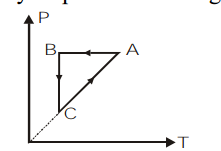$A)$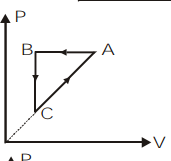$B)$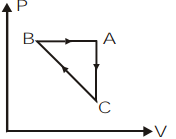$C)$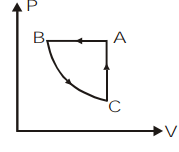$D)$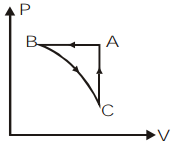Hint: We will use the ideal gas relation $PV\propto nT$ for analysis of all three processes given in the $P-T$ graph and convert the data into $P-V$ graph. The given process $ABCA$ is a cyclic process but it is completing one cycle with 3 processes. So we will need to convert each process separately.

The given process $ABCA$ completes one cycle by undergoing 3 cycles as $BC$, $CA$ and $AB$. We can plot the $P-V$ graph of the given process by analyzing the given $P-T$ graph individually for each process.
Let us take the first process $BC$ on the $P-T$ graph. We can observe that the temperature remains constant for this process and pressure is decreasing. So by the ideal gas equation, at constant temperature, $P \propto \dfrac{1}{V}$. So as the pressure is decreasing, the volume will increase.
Now, let us take the process $CA$ on the $P-T$ graph. Here, we can observe the temperature and pressure increasing as per the ideal gas relation $P \propto T$. But this relation is valid only at constant volume. So in the $P-V$ graph there will be no change in volume.
Then, we will consider the last process in this cyclic process which is $AB$. If we look at the $P-T$ graph of this process we can understand that pressure remains constant and temperature is decreasing. So, according to the ideal gas relation, at constant pressure, $V\propto T$. That means the volume will decrease. So in the $P-V$ graph the pressure remains constant and volume will decrease.
So, we can plot the $P-V$ graph of the given cyclic process $ABCA$ as,